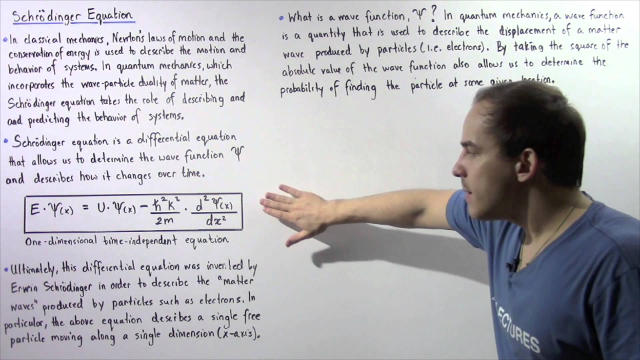# How do you teach Schrödinger’s equation to a 15 year old?Some 15-year olds can learn the Schrödinger equation from a textbook. But if the 15-year old is not mathematically sophisticated, you can’t expect to give them a full understanding of the Schrödinger equation in 15 minutes, but you can explain to them why the Schrödinger equation is needed and what it intuitively represents.

My attempt at an explanation would cover the following points, possibly omitting some math depending on whether the 15-year old knows any calculus:

• Physicists noticed that waves in pieces of string, sound waves in the air, and electromagnetic waves all seem to follow the same laws of wave motion. So they invented an equation called the “wave equation” that lets you predict what will happen to a wave in the future if you know what it looks like now.
• We can do experiments with wave tanks, tuning forks, and double-slit apparatuses to show that all kinds of waves interfere with each other, producing patterns with periodic nodes (areas where the wave is not detected at all).
• The double-slit experiment can be done with electrons, and the same interference pattern shows up, which proves that an electron beam is wave-like in the same way as a light beam. By comparing the electron interference patterns with the light interference patterns, we can prove that the more momentum an electron has, the shorter its wavelength. Ultimately, scientists discovered that every type of particle also has wave-like behaviour that follows the same law.
• We need an equation to describe the wave-like behaviour of particles. However, these particle waves are a bit different from water waves, since they involve complex numbers rather than real numbers like the height of the water in the wave. So the usual wave equation does not apply to particles. So physicists invented a different equation that seems to accurately describe the wave-like behaviour of particles, which is called the Schrödinger equation.
• The left-hand side of the Schrödinger equation tells us how to calculate the energy of the particle. The derivative on the left-hand side calculates the wavelength of the particle and therefore its momentum, then uses the momentum to calculate the kinetic energy. The left-hand side also adds in the particle’s potential energy. The derivative on the right-hand side calculates the frequency of the particle. So the Schrödinger equation relates the particle’s momentum with its energy, and also relates the particle’s wavelength with its frequency.

Source: https://www.quora.com/How-do-you-teach-Schr%C3%B6dinger%E2%80%99s-equation-to-a-15-year-old